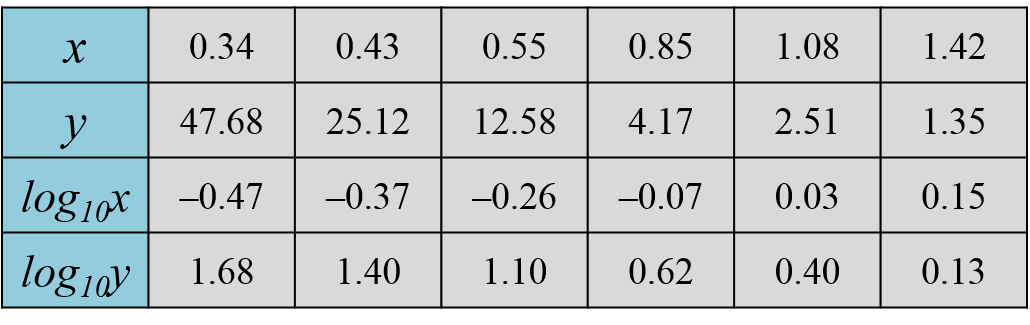# SPM Additional Mathematics 2019, Paper 2 (Question 11)

Question 11:
Use a graph paper to answer this question.
Table 2 shows the values of two variables, x and y, obtained from an experiment. The variables x and y are related by the equation $y=\frac{a}{{}^{b}\sqrt{x}},$ where a and b are constants.Table 2

(a) Based on Table 2, construct a table for the values of log10x and log10y. [2 marks]

(b) Plot log10y against log10x, using a scale of 2 cm to 0.1 unit on the X-axis and 2 cm to 0.2 unit on the Y-axis.
Hence, draw the line of best fit. [3 marks]

(c) Using the graph in 11(b), find the value of [5 marks]
(i) a,
(ii) b.

Solution:
(a)(b)(c)

(c)(i)

(c)(ii)